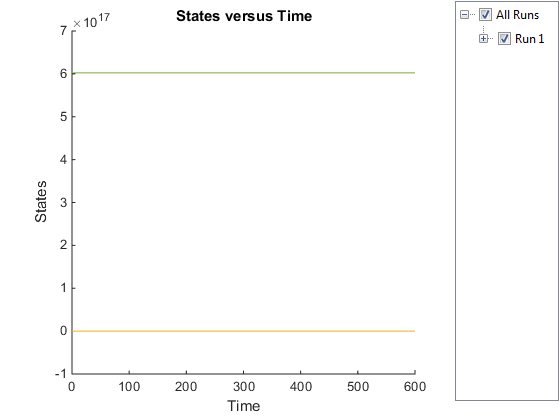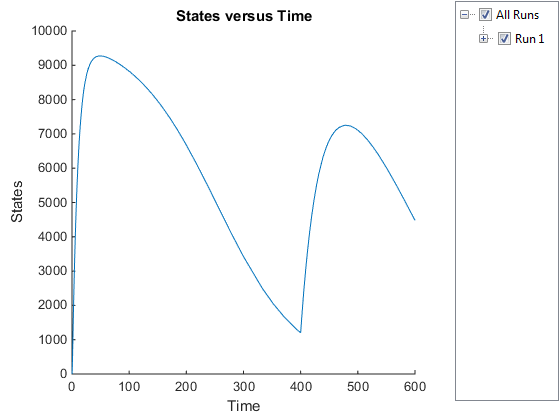## Create and Simulate a Model with a Custom Function

This example shows how to create a custom function and incorporate it in model simulation.

### Overview

#### Prerequisites for the Example

This example assumes that you have a working knowledge of:

• MATLAB® app

• Creating and saving MATLAB programs

This example uses the model described in Model of the Yeast Heterotrimeric G Protein Cycle.

This table shows the reactions used to model the G protein cycle and the corresponding rate parameters (rate constants) for each reaction. For reversible reactions, the forward rate parameter is listed first.

No.NameReaction1Rate Parameters
1Receptor-ligand interaction`L + R <-> RL``kRL`, `kRLm`
2Heterotrimeric G protein formation`Gd + Gbg -> G``kG1`
3G protein activation`RL + G -> Ga + Gbg + RL``kGa`
4Receptor synthesis and degradation`R <-> null``kRdo`, `kRs`
5Receptor-ligand degradation`RL -> null``kRD1`
6G protein inactivation`Ga -> Gd``kGd`
1 Legend of species: L = ligand (alpha factor), R = alpha-factor receptor, Gd = inactive G-alpha-GDP, Gbg = free levels of G-beta:G-gamma complex, G = inactive Gbg:Gd complex, Ga = active G-alpha-GTP

#### Assumptions of the Example

This example assumes that:

• An inhibitor (`Inhib` species) slows the inactivation of the active G protein (reaction 6 above, ```Ga –> Gd```).

• The variation in the amount of inhibitor (`Inhib` species) is defined in a custom function, `inhibvalex`.

• The inhibitor (`Inhib` species) affects the reaction by changing the amount of rate parameter `kGd`.

This example shows how to create and call a custom function in a SimBiology® expression. Specifically, it shows how to use a custom function in a rule expression.

#### About Using Custom Functions in SimBiology Expressions

You can use custom functions in:

• Reaction rate expressions (`ReactionRate` property)

• Rule expressions (`Rule` property)

• Event expressions (`EventFcns` property or `Trigger` property)

The requirements for using custom functions in SimBiology expressions are:

• Create a custom function. For more information, see `function`.

• Change the current folder to the folder containing your custom MATLAB file. Do this by using the `cd` command or by using the Current Folder field in the MATLAB desktop toolbar. Alternatively, add the folder containing your file to the search path. Do this by using the `addpath` command or see Change Folders on Search Path.

• Call the custom function in a SimBiology reaction, rule, or event expression.

Tip

If your rule or reaction rate expression is not continuous and differentiable, see Using Events to Address Discontinuities in Rule and Reaction Rate Expressions before simulating your model.

### Create a Custom Function

The following procedure creates a custom function, `inhibvalex`, which lets you specify how the inhibitor amount changes over time. The inputs are time, the initial amount of inhibitor, and a parameter that governs the amount of inhibitor. The output of the function is the amount of inhibitor.

In the MATLAB desktop, select File > New > Script, to open the MATLAB Editor.

Copy and paste the following function declaration:

```% inhibvalex.m function Cp = inhibvalex(t, Cpo, kel) % This function takes the input arguments t, Cpo, and kel % and returns the value of the inhibitor Cp. % You can later specify the input arguments in a % SimBiology rule expression. % For example in the rule expression, specify: % t as time (a keyword recognized as simulation time), % Cpo as a parameter that represents the initial amount of inhibitor, % and kel as a parameter that governs the amount of inhibitor. if t < 400 Cp = Cpo*exp(-kel*(t)); else Cp = Cpo*exp(-kel*(t-400)); end```

Save the file (name the file `inhibvalex.m`) in a directory that is on the MATLAB search path, or to a directory that you can access.

If the location of the file is not on the MATLAB search path, change the working directory to the file location.

Load the `gprotein` example project, which includes the variable `m1`, a model object:

`sbioloadproject gprotein`

The `m1` model object appears in the MATLAB Workspace.

### Add the Custom Function to the Example Model

The following procedure creates a rule expression that calls the custom function, `inhibvalex`, and specifies the three input values to this function.

Add a repeated assignment rule to the model that specifies the three input values to the custom function, `inhibvalex`:

```rule1 = addrule(m1, 'Inhib = inhibvalex(time, Cpo, Kel)',... 'repeatedAssignment');```

The `time` input is a SimBiology keyword recognized as simulation time

Create the two parameters used by the `rule1` rule and assign values to them:

```p1 = addparameter(m1, 'Cpo', 250); p2 = addparameter(m1, 'Kel', 0.01); ```

Create the species used by the `rule1` rule:

`s1 = addspecies(m1.Compartments, 'Inhib');`

### Define a Rule to Change Parameter Value

The value of rate parameter `kGd` is affected by the amount of inhibitor present in the system. Add a rule to the model to describe this action, but first change the `ConstantValue` property of the parameter `kGd` so that it can be varied by a rule.

Change the `ConstantValue` property of the `kGd` parameter to `false`.

```p3 = sbioselect(m1, 'Type', 'parameter', 'Name', 'kGd'); p3.ConstantValue = false;```

Add a repeated assignment rule to the model to define how the `kGd` parameter is affected by the `Inhib` species.

`rule2 = addrule(m1, 'kGd = 1/Inhib', 'repeatedAssignment');`

### Add an Event to Reset the Solver at a Discontinuity and Simulate the Model

The custom function, `inhibvalex`, introduces a discontinuity in the model when time = 400. To ensure accurate simulation results, add an event to the model to reset the solver at the time of the discontinuity. Set the event to trigger at the time of the discontinuity (time = 400). The event does not need to modify the model, so create an event function that multiplies a species value by 1.

`addevent(m1, 'time>=400', 'G=1*G');`

Configure the simulation settings (`configset object`) for the `m1` model object to log all states during the simulation.

```cs = getconfigset(m1); cs.RuntimeOptions.StatesToLog = 'all'; ```

Simulate the model.

`simDataObj = sbiosimulate(m1);`

Plot the results.

```sbioplot(simDataObj); ```The plot does not show the species of interest due to the wide range in species amounts/concentrations.

Plot only the species of interest. `Ga`.

```GaSimDataObj = selectbyname(simDataObj,'Ga'); sbioplot(GaSimDataObj); ```Notice the change in the profile of species `Ga` at time = `400` seconds (simulation time). This is the time when the inhibitor amount is changed to reflect the re-addition of inhibitor to the model.

Plot only the inhibitor (`Inhib` species).

```InhibSimDataObj = selectbyname(simDataObj,'Inhib'); sbioplot(InhibSimDataObj) ```